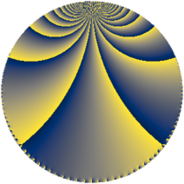# Properties

 Label 252.4.bLevel $252$ Weight $4$ Character orbit 252.b Rep. character $\chi_{252}(55,\cdot)$ Character field $\Q$ Dimension $58$ Newform subspaces $7$ Sturm bound $192$ Trace bound $7$

# Learn more

## Defining parameters

 Level: $$N$$ $$=$$ $$252 = 2^{2} \cdot 3^{2} \cdot 7$$ Weight: $$k$$ $$=$$ $$4$$ Character orbit: $$[\chi]$$ $$=$$ 252.b (of order $$2$$ and degree $$1$$) Character conductor: $$\operatorname{cond}(\chi)$$ $$=$$ $$28$$ Character field: $$\Q$$ Newform subspaces: $$7$$ Sturm bound: $$192$$ Trace bound: $$7$$ Distinguishing $$T_p$$: $$5$$, $$11$$, $$19$$

## Dimensions

The following table gives the dimensions of various subspaces of $$M_{4}(252, [\chi])$$.

Total New Old
Modular forms 152 62 90
Cusp forms 136 58 78
Eisenstein series 16 4 12

## Trace form

 $$58q + q^{2} - 7q^{4} - 23q^{8} + O(q^{10})$$ $$58q + q^{2} - 7q^{4} - 23q^{8} - 67q^{14} - 43q^{16} - 182q^{22} - 1086q^{25} - 199q^{28} - 140q^{29} - 559q^{32} - 516q^{37} + 454q^{44} - 250q^{46} - 694q^{49} + 1229q^{50} + 20q^{53} + 133q^{56} + 66q^{58} + 2153q^{64} - 1008q^{65} - 848q^{70} - 498q^{74} - 1324q^{77} + 2320q^{85} - 1138q^{86} + 346q^{88} - 1918q^{92} + 1625q^{98} + O(q^{100})$$

## Decomposition of $$S_{4}^{\mathrm{new}}(252, [\chi])$$ into newform subspaces

Label Dim. $$A$$ Field CM Traces $q$-expansion
$$a_2$$ $$a_3$$ $$a_5$$ $$a_7$$
252.4.b.a $$2$$ $$14.868$$ $$\Q(\sqrt{-7})$$ $$\Q(\sqrt{-7})$$ $$-5$$ $$0$$ $$0$$ $$0$$ $$q+(-3+\beta )q^{2}+(7-5\beta )q^{4}+(-7+14\beta )q^{7}+\cdots$$
252.4.b.b $$4$$ $$14.868$$ $$\Q(\sqrt{-2}, \sqrt{7})$$ $$\Q(\sqrt{-21})$$ $$0$$ $$0$$ $$0$$ $$0$$ $$q-2\beta _{1}q^{2}-8q^{4}+\beta _{2}q^{5}-7\beta _{3}q^{7}+\cdots$$
252.4.b.c $$4$$ $$14.868$$ $$\Q(i, \sqrt{7})$$ $$\Q(\sqrt{-7})$$ $$0$$ $$0$$ $$0$$ $$0$$ $$q-\beta _{3}q^{2}+(-2-5\beta _{2})q^{4}+(-7+14\beta _{2}+\cdots)q^{7}+\cdots$$
252.4.b.d $$8$$ $$14.868$$ $$\mathbb{Q}[x]/(x^{8} - \cdots)$$ None $$8$$ $$0$$ $$0$$ $$0$$ $$q+(1-\beta _{2})q^{2}+(-2-\beta _{2}+\beta _{4}+\beta _{5}+\cdots)q^{4}+\cdots$$
252.4.b.e $$12$$ $$14.868$$ $$\mathbb{Q}[x]/(x^{12} - \cdots)$$ None $$-1$$ $$0$$ $$0$$ $$-10$$ $$q-\beta _{1}q^{2}+\beta _{2}q^{4}+(-\beta _{1}-\beta _{8})q^{5}+\cdots$$
252.4.b.f $$12$$ $$14.868$$ $$\mathbb{Q}[x]/(x^{12} - \cdots)$$ None $$-1$$ $$0$$ $$0$$ $$10$$ $$q-\beta _{2}q^{2}+\beta _{3}q^{4}+(\beta _{2}-\beta _{8})q^{5}+(1+\cdots)q^{7}+\cdots$$
252.4.b.g $$16$$ $$14.868$$ $$\mathbb{Q}[x]/(x^{16} - \cdots)$$ None $$0$$ $$0$$ $$0$$ $$0$$ $$q+\beta _{2}q^{2}+(3+\beta _{5})q^{4}+\beta _{11}q^{5}+(1+\cdots)q^{7}+\cdots$$

## Decomposition of $$S_{4}^{\mathrm{old}}(252, [\chi])$$ into lower level spaces

$$S_{4}^{\mathrm{old}}(252, [\chi]) \cong$$ $$S_{4}^{\mathrm{new}}(28, [\chi])$$$$^{\oplus 3}$$$$\oplus$$$$S_{4}^{\mathrm{new}}(84, [\chi])$$$$^{\oplus 2}$$